Home > CC4 > Chapter 11 > Lesson 11.1.3 > Problem11-37

11-37.
1. Solve this system of equations: Homework Help ✎

y =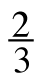x − 4
2x − 3y = 10

1. What does your solution tell you about the relationship between the lines?

2. Solve the second equation for y.

3. Does the slope of each line confirm your statement in part (a)? Explain how.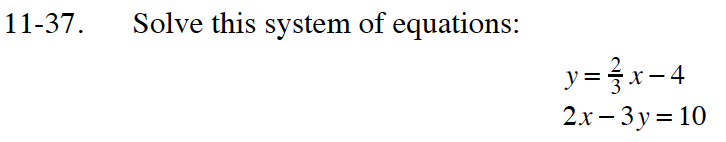Try using the Substitution method.

No solution.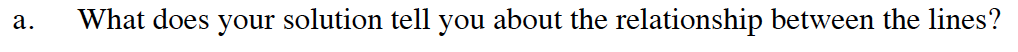When a solution exists it represents the point where the lines intersect.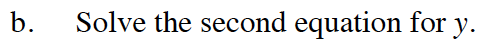$y=-\frac{10}{3}+\frac{2}{3}x \text{ or }y=\frac{2}{3}x-\frac{10}{3}$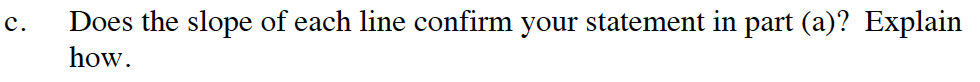What can you say about lines that have the same slopes?